Function Repository Resource:

# NormalVector

Compute the normal vector of a curve

Contributed by: Wolfram Staff (original content by Alfred Gray)
 ResourceFunction["NormalVector"][c,t] computes the normal vector for a curve c parametrized by t.

## Details and Options

The normal vector, or simply the "normal" to a curve, is a vector perpendicular to a curve or surface at a given point. It is usually represented by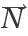.

## Examples

### Basic Examples (2)

A unit speed helix:

 In:=Compute the normal vector:

 In:=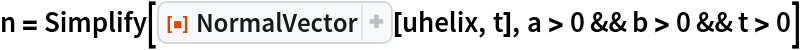Out=### Scope (3)

The normal vector of a figure-eight curve:

 In:=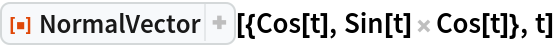Out=In:=Out=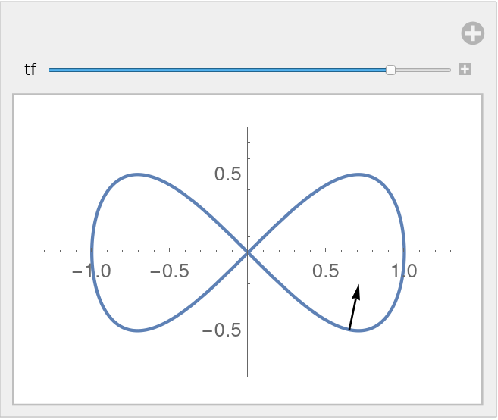Plot a set of normal vectors:

 In:=Out=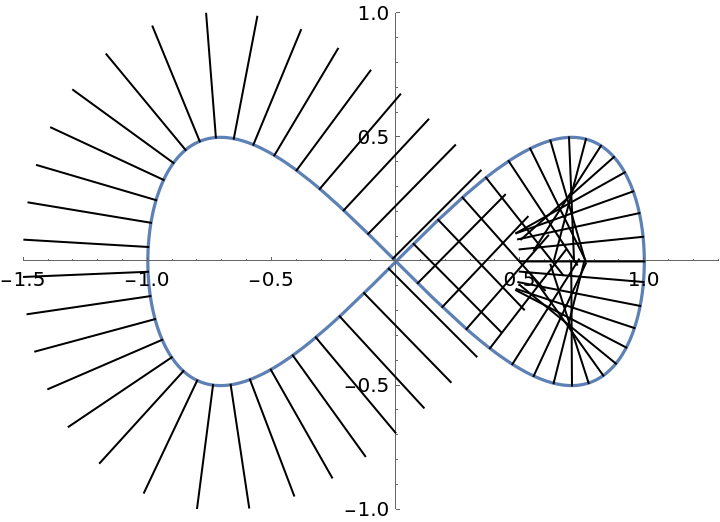Define a helix curve:

 In:=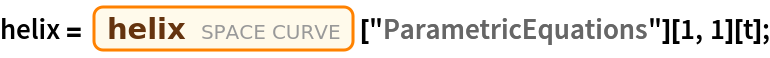Compute the normal vector:

 In:=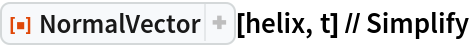Out=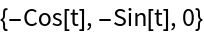The binormal vector, via the resource function BinormalVector:

 In:=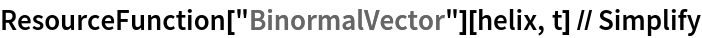Out=The normal plane is spanned by the normal vector and the binormal vector. It can be computed with the resource function NormalPlane:

 In:=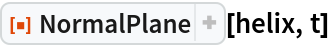Out=The normal plane and the normal vector along the helix:

 In:=Out=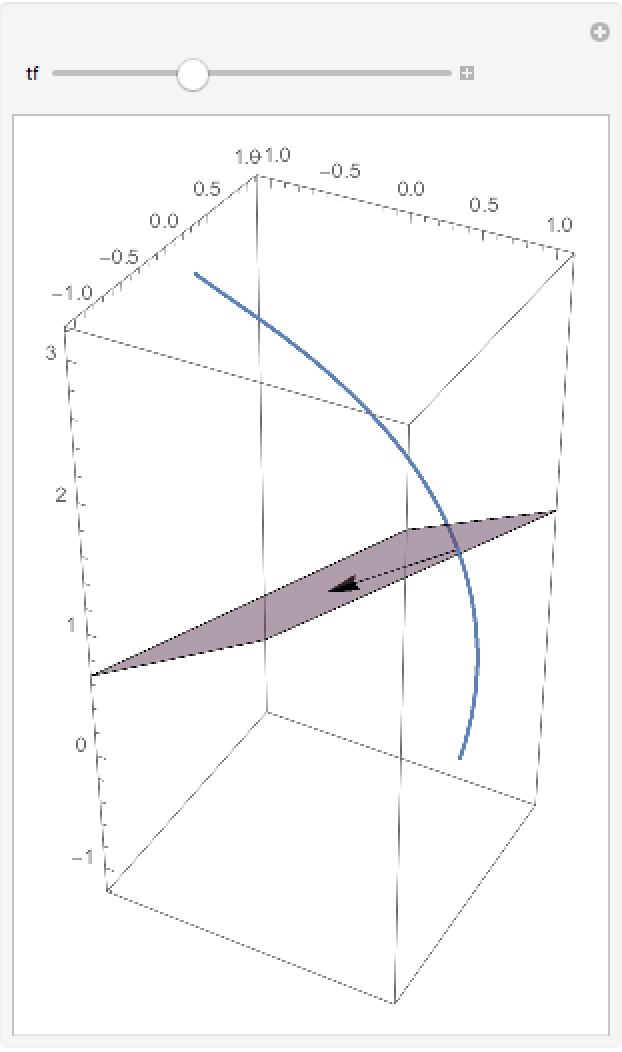### Properties and Relations (2)

The definition of Viviani's curve:

 In:=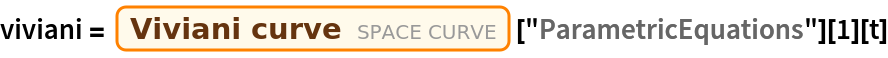Out=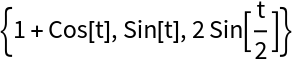Plot the curve:

 In:=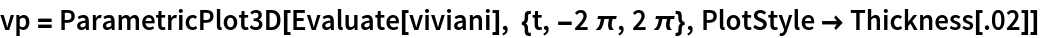Out=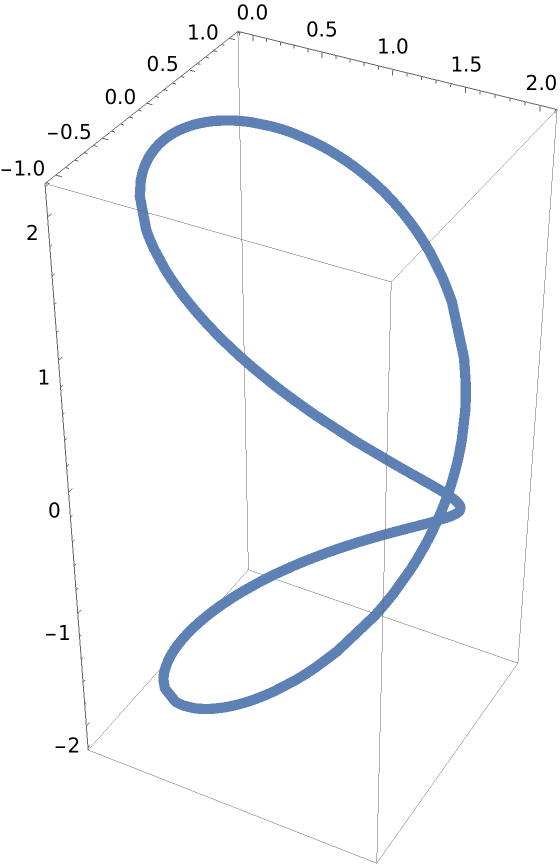The normal surface associated to a curve is generated by its normal vector field. It can be computed with the resource function NormalSurface:

 In:=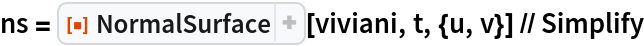Out=Plot the normal surface:

 In:=Out=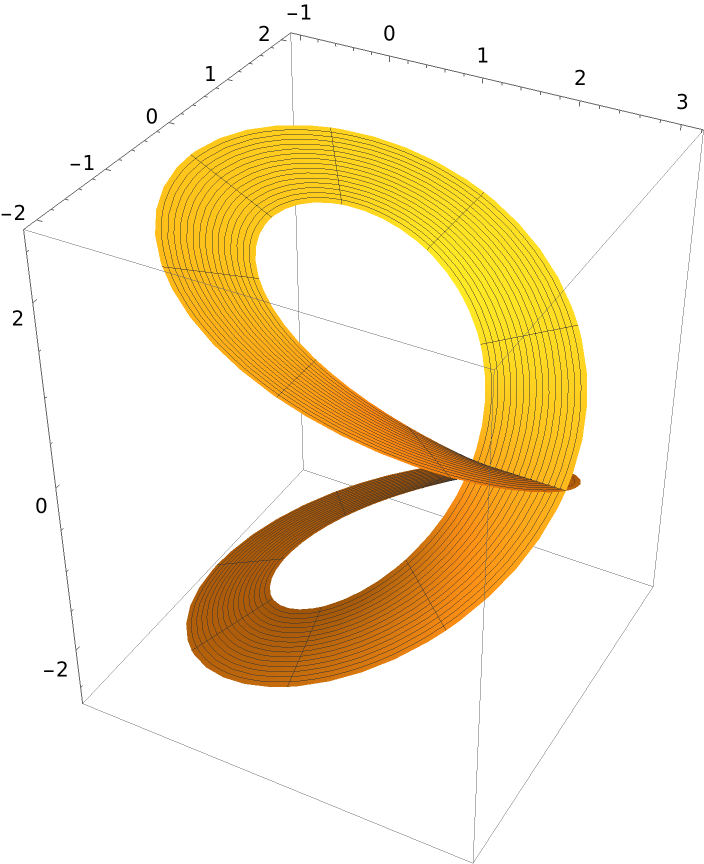A unit speed helix:

 In:=Compute the normal vector:

 In:=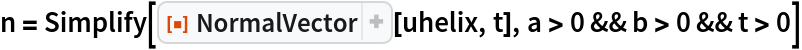Out=Compute the curvature with the resource function Curvature:

 In:=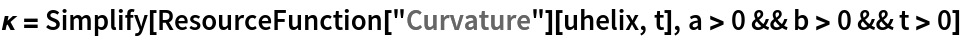Out=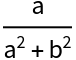Compute the torsion with the resource function CurveTorsion:

 In:=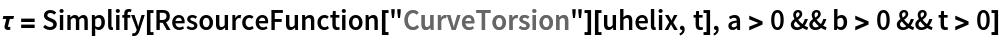Out=Compute the tangent vector with the resource function TangentVector:

 In:=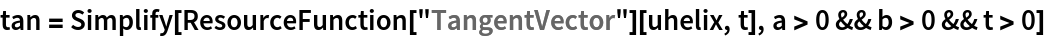Out=Compute the binormal vector with the resource function BinormalVector:

 In:=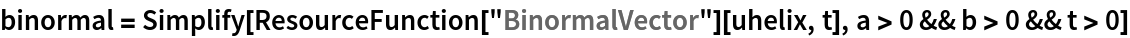Out=Compute the following quantity:

 In:=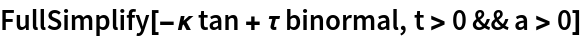Out=The previous expression is the derivative of the normal:

 In:=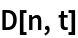Out=Using FrenetSerretSystem, the normal vector is the second entry of the second List:

 In:=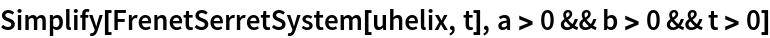Out=Enrique Zeleny

## Version History

• 2.0.0 – 30 April 2020
• 1.0.0 – 28 April 2020